Harmonic Progression - Examples (with Solutions), Algebra, Quantitative Aptitude

# Harmonic Progression - Examples (with Solutions), Algebra, Quantitative Aptitude | General Aptitude for GATE - Mechanical Engineering

 1 Crore+ students have signed up on EduRev. Have you?

Harmonic Progression (H.P.)

1. A series of quantities is said to be in a harmonic progression when their reciprocals are in arithmetic progression.

2. e.g.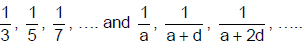are in HP as their reciprocals

3. 3, 5, 7, …, and a, a + d, a + 2d….…. are in AP.

nth term of HP

1. Find the nth term of the corresponding AP and then take its reciprocal.

2. If the HP be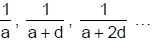3. Then the corresponding AP is a, a + d, a + 2d, ……

4. Tn of the AP is a + (n - 1) d
5. Tnth of the HP is ……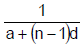6. In order to solve a question on HP, one should form the corresponding AP.

A comparison between AP and GP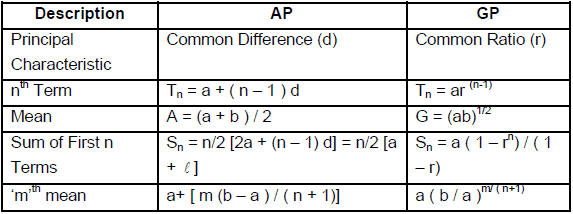Arithmetic - Geometric progression
a + (a + d)r + (a + 2d)r+ (a + 3d)r3 + ……………. Is the form of Arithmetic geometric progression (A.G.P).
One part of the series is in Arithmetic progression and other part is a Geometric progression.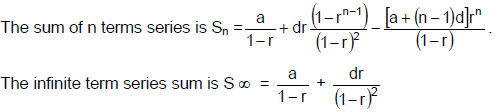Arithmetic geometric series can be solved as explained in the example below:

Relation between AM, GM and HM:

For two positive numbers a and b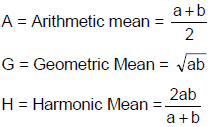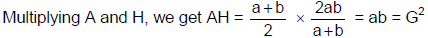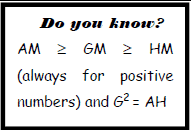This mean A, G, H are in G.P.
Verifying for numbers 1, 2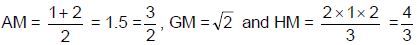Hence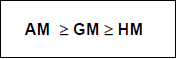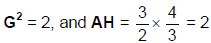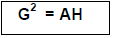Toolkit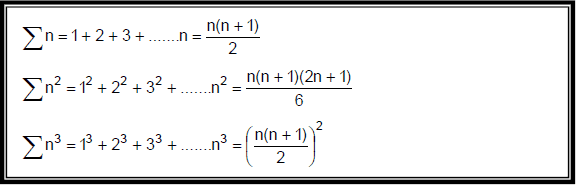Ex.1 Find the sum of 1 + 2x + 3x2 + 4x3 + … ∞

Sol. The given series in an arithmetic-geometric series whose corresponding A.P. and G.P. are 1, 2, 3, 4,…
and 1, x, x2, x3, … respectively. The common ratio of the G.P. is x. Let S denote the sum of the given
series.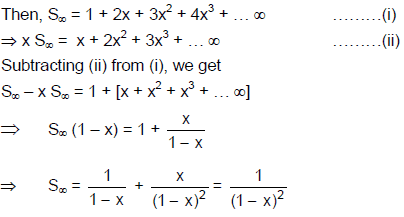Ex.2 If the first item of an A.P is 12, and 6th term is 27. What is the sum of first 10 terms?

Sol.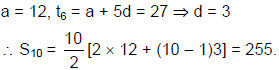Ex.3 If the fourth & sixth terms of an A.P are 6.5 and 9.5. What is the 9th term of that A.P?

Sol.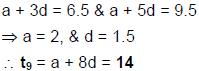Ex.4 What is the arithmetic mean of first 20 terms of an A.P. whose first term is 5 and 4th term is 20?

Sol.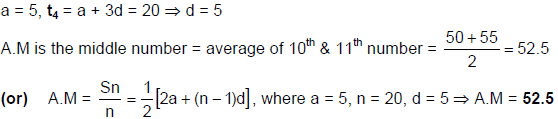Ex.5 The first term of a G.P is half of its fourth term. What is the 12th term of that G.P, if its sixth term
is 6

Sol.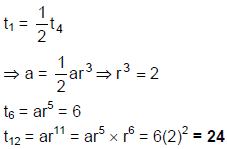Ex.6 If the first and fifth terms of a G.P are 2 and 162. What is the sum of these five terms?

Sol.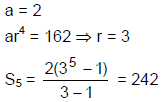Ex.7 What is the value of r + 3r2 + 5r3 + - - - - -

Sol.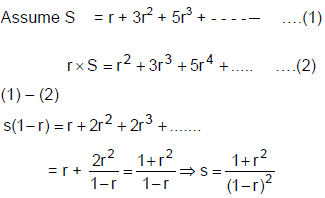Ex.8 The first term of a G.P. 2 and common ratio is 3. If the sum of first n terms of this G.P is greater
than 243 then the minimum value of ‘n’ is

Sol.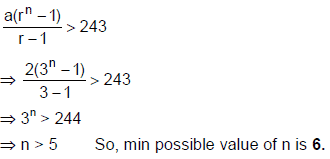Ex.9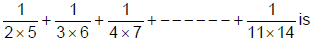Sol.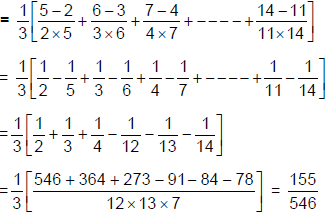Ex.10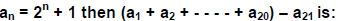Sol.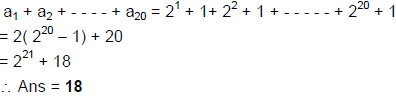The document Harmonic Progression - Examples (with Solutions), Algebra, Quantitative Aptitude | General Aptitude for GATE - Mechanical Engineering is a part of the Mechanical Engineering Course General Aptitude for GATE.
All you need of Mechanical Engineering at this link: Mechanical Engineering
FAQs on Harmonic Progression - Examples (with Solutions), Algebra, Quantitative Aptitude | General Aptitude for GATE - Mechanical Engineering
 1. What is Harmonic Progression?Ans. Harmonic Progression is a sequence of numbers in which the reciprocal of each term is in arithmetic progression. For example, 1, 1/2, 1/3, 1/4... is a harmonic progression because its reciprocals 1, 2, 3, 4... are in arithmetic progression.
 2. How do you find the nth term of a harmonic progression?Ans. The nth term of a harmonic progression can be found using the formula: a(n) = 1/(1/d + (n-1) * (1/a - 1/d)) where a is the first term and d is the common difference between the reciprocals of the terms.
 3. What are some applications of Harmonic Progression?Ans. Harmonic Progression has several applications in fields such as physics, engineering, and finance. In physics, it is used to calculate the resonant frequency of an oscillating system. In engineering, it is used to design electrical circuits and calculate the capacitance of capacitors. In finance, it is used to calculate the average rate of return on investments.
 4. How can Harmonic Progression be differentiated from Arithmetic Progression and Geometric Progression?Ans. Arithmetic Progression is a sequence of numbers in which each term is obtained by adding a fixed number to the preceding term. Geometric Progression is a sequence of numbers in which each term is obtained by multiplying the preceding term by a fixed number. Harmonic Progression is a sequence of numbers in which the reciprocal of each term is in arithmetic progression.
 5. What are some common problems that can be solved using Harmonic Progression?Ans. Harmonic Progression can be used to solve problems related to time, speed, and distance. For example, if two people start at the same place and walk towards each other at speeds of x and y km/h respectively, the time taken for them to meet can be calculated using Harmonic Progression. Similarly, it can be used to calculate the average speed of a journey when the distance covered in each leg of the journey is different.

## General Aptitude for GATE

73 videos|86 docs|108 tests

## General Aptitude for GATE

73 videos|86 docs|108 tests

Track your progress, build streaks, highlight & save important lessons and more!(Scan QR code)

,

,

,

,

,

,

,

,

,

,

,

,

,

,

,

,

,

,

,

,

,

,

,

,

,

,

,

;